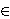### Maja Fošner and Nina Peršin

Faculty of logistics, University of Maribor, Mariborska cesta 7, 3000 Celje, Slovenia
e-mail: maja.fosner@uni-mb.si

Prušnikova 48, 2000 Maribor, Slovenia
e-mail: nina-persin@t-2.net

Abstract.   A classical result of Herstein asserts that any Jordan derivation on a prime ring of characteristic different from two is a derivation. It is our aim in this paper to prove the following result, which is in the spirit of Herstein's theorem. Let R be a prime ring with char(R)=0 or 4 < char(R), and let D:R → R be an additive mapping satisfying either the relation D(x3)=D(x2)x+x2D(x) or the relation D(x3)=D(x)x2+xD(x2) for all xR. In both cases D is a derivation.

2010 Mathematics Subject Classification.   16W10, 46K15, 39B05.

Key words and phrases.   Prime ring, semiprime ring, derivation, Jordan derivation, Jordan triple derivation, functional identity.

Full text (PDF) (access from subscribing institutions only)

DOI: 10.3336/gm.48.1.06

References:

1. K. I. Beidar, M. Brešar, M. A. Chebotar and W. S. Martindale 3rd, On Herstein's Lie map conjectures. II, J. Algebra 238 (2001), 239-264.
MathSciNet     CrossRef

2. K. I. Beidar and Y. Fong, On additive isomorphisms of prime rings preserving polynomials, J. Algebra 217 (1999), 650-667.
MathSciNet     CrossRef

3. M. Brešar, Jordan derivations on semiprime rings, Proc. Amer. Math. Soc. 104 (1988), 1003-1006.
MathSciNet     CrossRef

4. M. Brešar, Jordan mappings of semiprime rings, J. Algebra 127 (1989), 218-228.
MathSciNet     CrossRef

5. M. Brešar, Functional identities: a survey, Contemp. Math. 259 (2000), 93-109.
MathSciNet     CrossRef

6. M. Brešar, M. A. Chebotar and W. S. Martindale 3rd, Functional identities, Birkhäuser Verlag, Basel, 2007.
MathSciNet

7. M. Brešar and J. Vukman, Jordan derivations on prime rings, Bull. Austral. Math. Soc. 37 (1988), 321-323.
MathSciNet     CrossRef

8. M. Brešar and J. Vukman, Jordan (θ ,φ)-derivations, Glas. Mat. Ser. III 26(46) (1991), 13-17.
MathSciNet

9. Cheng-Kai Liu and Wen-Kwei Shiue, Generalized Jordan triple (θ ,φ)-derivations on semiprime rings, Taiwanese J. Math. 11 (2007), 1397-1406.
MathSciNet

10. J. M. Cusack, Jordan derivations on rings, Proc. Amer. Math. Soc. 53 (1975), 321-324.
MathSciNet     CrossRef

11. I. N. Herstein, Jordan derivations of prime rings, Proc. Amer. Math. Soc. 8 (1957), 1104-1110.
MathSciNet     CrossRef

12. L. Rowen, Some results on the center of a ring with polynomial identity, Bull. Amer. Math. Soc. 79 (1973), 219-223.
MathSciNet     CrossRef

13. J. Vukman, On derivations of standard operator algebras and semisimple H*-algebras, Studia Sci. Math. Hungar. 44 (2007), 57-63.
MathSciNet     CrossRef

14. J. Vukman, Some remarks on derivations in semiprime rings and standard operator algebras, Glas. Mat. Ser. III 46(66) (2011), 43-48.
MathSciNet     CrossRef

15. J. Vukman, I. Kosi-Ulbl and D. Eremita, On certain equations in rings, Bull. Austral. Math. Soc. 71 (2005), 53-60.
MathSciNet     CrossRef